Get instant live expert help with Excel or Google Sheets“My Excelchat expert helped me in less than 20 minutes, saving me what would have been 5 hours of work!”

#### Post your problem and you'll get expert help in seconds

Your message must be at least 40 characters
Our professional experts are available now. Your privacy is guaranteed.

# Sum race time splitsRead time: 20 minutes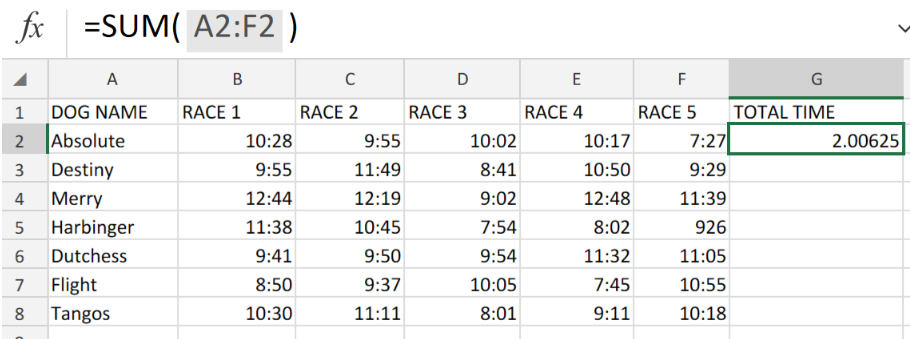Figure 1. Sum Race Time Splits in Excel.

Let us assume that we are required to sum up different race time splits that are the results of races between several competitors, we are going to use the Excel SUM function to achieve this.

## Generic Formula

`=SUM(range)`

The Excel SUM function determines the total of different time splits made up of hours, minutes, and seconds.

## How to use the Excel SUM function

To use the SUM function in Excel, use the following 3 simple steps.

1. Collect the different time splits from the races and arrange them into labeled columns of our worksheet.

See example illustrated below;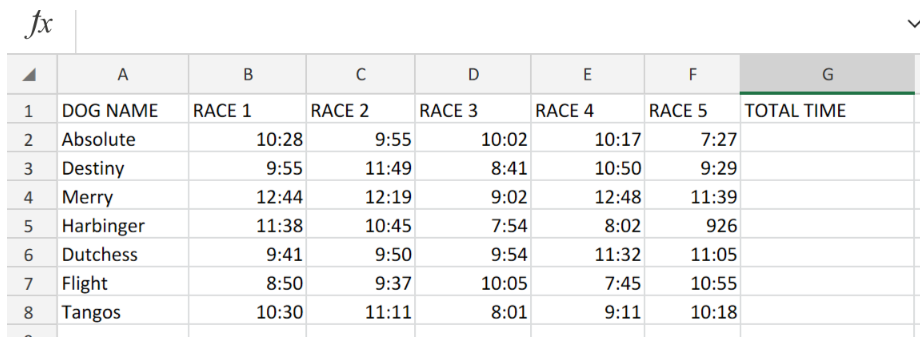Figure 2. Sum Race Time Splits in Excel.

Be sure to provide empty cells for Excel to determine the TOTAL TIME taken.

1. In our worksheet example above, the SUM formula in cell G2 is as follows;.

`=SUM(A2:F2)`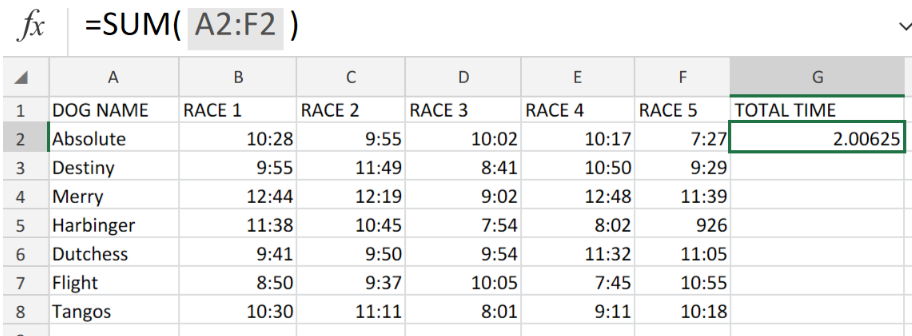Figure 3. Sum Race Time Splits in Excel.

1. Copy the modified version of the SUM formula into the other cells in the TOTAL TIME column to get the other results.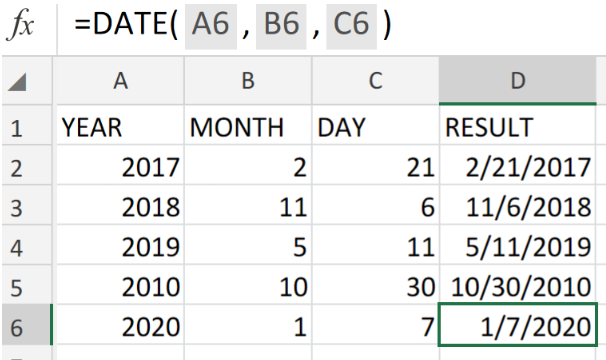Figure 4. Sum Race Time Splits in Excel.

However, when working with times, care must be taken to input time values with the right syntax/format to display the ideal results.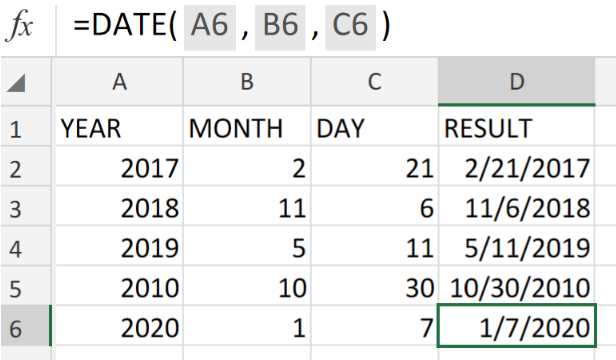Figure 5. Final Result.

## Instant Connection to an Expert through our Excelchat Service:

Our live Excelchat Service is here for you. We have Excel Experts available 24/7 to answer any Excel questions you may have. Guaranteed connection within 30 seconds and a customized solution for you within 20 minutes.

### Did this post not answer your question? Get a solution from connecting with the expert.Another blog reader asked this question today on Excelchat:
Related blogs## Subscribe to Excelchat.coAnother blog reader asked this question today on Excelchat: